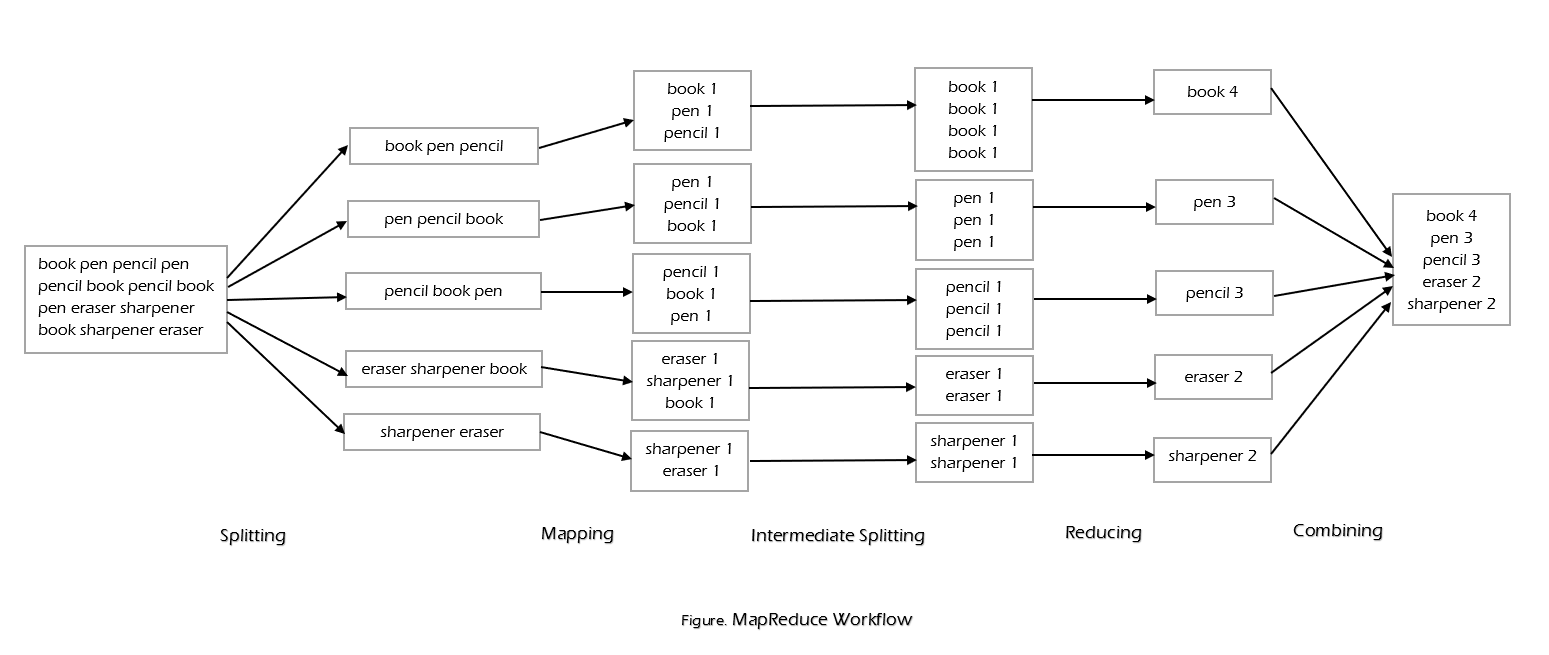###### Summary -

In this topic, we described about the Hadoop Implementation with detailed example.

Applications data processing on Hadoop are written using the MapReduce paradigm. A MapReduce usually splits the input data-set into independent chunks, which are processed by the map tasks in a completely parallel manner. The framework sorts the outputs of maps, which are then input to reduce the tasks.

Both input and output of the job are stored in the file system. MapReduce applications specify the input/output locations and supply MapReduce functions via implementation of appropriate Hadoop interfaces such as Mapper and Reducer. MapReduce consists of two phases – Map and Reduce.

Map phase takes a set of data and converts it into another set of data, where individual elements are broken down into key pairs. Reduce phase, which takes the output from a map as an input and combines those data tuples into a smaller set of tuples. This chapter explains the MapReduce implementation in Hadoop. Let us discuss the same with simple word count example.

Input: book pen pencil pen pencil book pencil book pen eraser sharpener book sharpener. Save the input as input.txt and place it in the Hadoop library.

``````\$vim input.txt
book pen pencil pen pencil book pencil book
pen eraser sharpener book sharpener
\$``````
``````\$hadoop fs -mkdir -p /user/user-name/wc/input

#### Work Flow:Workflow of MapReduce consists of five steps -

1. Splitting: The splitting parameter can be any character which is specified.
2. Mapping: The mapping takes a set of data and converts it into tuples (Key-Value pair).
3. Intermediate Splitting: The process is to combine the same tubles from different clusters to provide an input to Reduce.
4. Reduce: The reduce will group by phase.
5. Combining: The last phase where the phases will be combined together to provide the output.

The only prerequisite to write the wordcount program, in java is Hadoop should install on your system with java idk.

Writing all of the above steps are not required and the need to write the splitting parameter, Map function logic, and Reduce function logic. The remaining steps will execute automatically.

Step-1: Write the Program with java code

``````package org.example.wordcount;
import java.io.IOException;
public class WordcountExample {
public int run(String[] args) throws Exception {
if (args.length != 2) {
System.out.println("Usage: [input] [output]");
System.exit(-1);
}
Job jobex = jobex.getInstance(getConf());
jobex.setJobName("wordcount");
jobex.setJarByClass(WordcountDriver.class);
jobex.setOutputKeyClass(Text.class);
jobex.setOutputValueClass(IntWritable.class);
jobex.setMapperClass(WordcountExampleMapper.class);
jobex.setCombinerClass(WordcountExampleReducer.class);
jobex.setReducerClass(WordcountExampleReducer.class);
jobex.setInputFormatClass(TextInputFormat.class);
jobex.setOutputFormatClass(TextOutputFormat.class);
Path inputFilePath = new Path(args);
Path outputFilePath = new Path(args);
/* This line is to accept the input recursively */
FileInputFormat.setInputDirRecursive(job, true);
FileOutputFormat.setOutputPath(job, outputFilePath);
/*  Delete output filepath if already exists */
FileSystem fs = FileSystem.newInstance(getConf());
if (fs.exists(outputFilePath)) {
fs.delete(outputFilePath, true);
}
return jobex.waitForCompletion(true) ? 0: 1;
}
}
public class WordcountExampleMapper extends
Mapper<LongWritable, Text, Text, IntWritable> {
private final static IntWritable one = new IntWritable(1);
private Text word = new Text();
public void map(LongWritable key, Text value, Context context)
throws IOException, InterruptedException {
String line = value.toString();
StringTokenizer tokenizer = new StringTokenizer(line);
while (tokenizer.hasMoreTokens()) {
Text singleword = new Text(word.toUpperCase().trim());
word.set(tokenizer.nextToken());
context.write(singleword, one);
}
}
}
public class WordcountExampleReducer extends
Reducer<Text, IntWritable, Text, IntWritable> {
private IntWritable totalWordCount = new IntWritable();
public void reduce(final Text key, final Iterable<IntWritable>
values,final Context context) throws IOException,
InterruptedException {
int totalcount = 0;
Iterator<IntWritable> iterator = values.iterator();
while (iterator.hasNext()) {
totalcount += iterator.next().get();
}
totalWordCount.set(totalcount);
context.write(key, totalWordCount);
}
}
``````

Step-2: Make the jar(WCExample.jar) file from the above code.

Step-3: Run the jar file.

``````\$ hadoop jar WCExample.jar  org.example.
wordcount.wordcountExample /user/user-name/wc/input
/user/user-name/wc/output``````

Step-4: Expected Result

``````\$ bin/hadoop fs -cat /user/ user-name
/wc/output/part-r-00000``````
```book 4
pen 3
pencil 3
sharpener 2
eraser 1
```